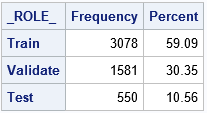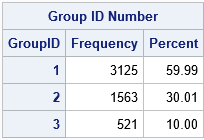In machine learning and other model building techniques, it is common to partition a large data set into three segments: training, validation, and testing.

• Training data is used to fit each model.
• Validation data is a random sample that is used for model selection. These data are used to select a model from among candidates by balancing the tradeoff between model complexity (which fit the training data well) and generality (but they might not fit the validation data). These data are potentially used several times to build the final model
• Test data is a hold-out sample that is used to assess final model and estimate its prediction error. It is only used at the end of the model-building process.

I've seen many questions about how to use SAS to split data into training, validation, and testing data. (A common variation uses only training and validation.) There are basically two approaches to partitioning data:

• Specify the proportion of observations that you want in each role. For each observation, randomly assign it to one of the three roles. The number of observations assigned to each role will be a multinomial random variable with expected value N pk, where N is the number of observations and pk (k = 1, 2, 3) is the probability of assigning an observation to the k_th role. For this method, if you change the random number seed you will usually get a different number of observations each role because the size is a random variable.
• Specify the number of observations that you want in each role and randomly allocate that many observations.

This article uses the SAS DATA step to accomplish the first task and uses PROC SURVEYSELECT to accomplish the second. I also discuss how to split data into only two roles: training and validation.

It is worth mentioning that many model-selection routines in SAS enable you to split data by using the PARTITION statement. Example include the "SELECT" procedures (GLMSELECT, QUANTSELECT, HPGENSELECT...) and the ADAPTIVEREG procedure. However, be aware that the procedures might ignore observations that have missing values for the variables in the model.

### Random partition into training, validation, and testing data

When you partition data into various roles, you can choose to add an indicator variable, or you can physically create three separate data sets. The following DATA step creates an indicator variable with values "Train", "Validate", and "Test". The specified proportions are 60% training, 30% validation, and 10% testing. You can change the values of the SAS macro variables to use your own proportions. The RAND("Table") function is an efficient way to generate the indicator variable.

```data Have; /* the data to partition */ set Sashelp.Heart; /* for example, use Heart data */ run;   /* If propTrain + propValid = 1, then no observation is assigned to testing */ %let propTrain = 0.6; /* proportion of trainging data */ %let propValid = 0.3; /* proportion of validation data */ %let propTest = %sysevalf(1 - &propTrain - &propValid); /* remaining are used for testing */   /* Randomly assign each observation to a role; _ROLE_ is indicator variable */ data RandOut; array p _temporary_ (&propTrain, &propValid); array labels \$ _temporary_ ("Train", "Validate", "Test"); set Have; call streaminit(123); /* set random number seed */ /* RAND("table") returns 1, 2, or 3 with specified probabilities */ _k = rand("Table", of p[*]); _ROLE_ = labels[_k]; /* use _ROLE_ = _k if you prefer numerical categories */ drop _k; run;   proc freq data=RandOut order=freq; tables _ROLE_ / nocum; run;```A shown by the output of PROC FREQ, the proportion of observations in each role is approximately the same as the specified proportions. For this random number seed, the proportions are 59.1%, 30.4%, and 10.6%. If you change the random number seed, you will get a different assignment of observations to roles and also a different proportion of data in each role.

The observant reader will notice that there are only two elements in the array of probabilities (p) that is used in the RAND("Table") call. This is intentional. The documentation for the RAND("Table") function states that if the sum of the specified probabilities is less than 1, then the quantity 1 – Σ pi is used as the probability of the last event. By specifying only two values in the p array, the same program works for partitioning the data into two pieces (training and validation) or three pieces (and testing).

### Create random training, validation, and testing data sets

Some practitioners choose to create three separate data sets instead of adding an indicator variable to the existing data. The computation is exactly the same, but you can use the OUTPUT statement to direct each observation to one of three output data sets, as follows:

```/* create a separate data set for each role */ data Train Validate Test; array p _temporary_ (&propTrain, &propValid); set Have; call streaminit(123); /* set random number seed */ /* RAND("table") returns 1, 2, or 3 with specified probabilities */ _k = rand("Table", of p[*]); if _k = 1 then output Train; else if _k = 2 then output Validate; else output Test; drop _k; run;```
```NOTE: The data set WORK.TRAIN has 3078 observations and 17 variables. NOTE: The data set WORK.VALIDATE has 1581 observations and 17 variables. NOTE: The data set WORK.TEST has 550 observations and 17 variables.```

This example uses the same random number seed as the previous example. Consequently, the three output data sets have the same observations as are indicated by the partition variable (_ROLE_) in the previous example.

### Specify the number of observations in each role

Instead of specifying a proportion, you might want to specify the exact number of observations that are randomly assigned to each role. The advantage of this technique is that changing the random number seed does not change the number of observations in each role (although it does change which observations are assigned to each role). The SURVEYSELECT procedure supports the GROUPS= option, which you can use to specify the number of observations.

The GROUPS= option requires that you specify integer values. For this example, the original data contains 5209 observations but 60% of 5209 is not an integer. Therefore, the following DATA step computes the number of observations as ROUND(N p) for the training and validation sets. These integer values are put into macro variables and used in the GROUPS= option on the PROC SURVEYSELECT statement. You can, of course, skip the DATA step and specify your own values such as groups=(3200, 1600, 409).

```/* Specify the sizes of the train/validation/test data from proportions */ data _null_; if 0 then set sashelp.heart nobs=N; /* N = total number of obs */ nTrain = round(N * &propTrain); /* size of training data */ nValid = round(N * &propValid); /* size of validataion data */ call symputx("nTrain", nTrain); /* put integer into macro variable */ call symputx("nValid", nValid); call symputx("nTest", N - nTrain - nValid); run;   /* randomly assign observations to three groups */ proc surveyselect data=Have seed=12345 out=SSOut groups=(&nTrain, &nValid, &nTest); /* if no Test data, use GROUPS=(&nTrain, &nValid) */ run;   proc freq data=SSOut order=freq; tables GroupID / nocum; /* GroupID is name of indicator variable */ run;```The training, validation, and testing groups contain 3125, 1563, and 521 observations, respectively. These numbers are the closest integer approximations to 60%, 30% and 10% of the 5209 observations. Notice that the output from the SURVEYSELECT procedure uses the values 1, 2, and 3 for the GroupID indicator variable. You can use PROC FORMAT to associate those numbers with labels such as "Train", "Validate", and "Test".

In summary, there are two basic programming techniques for randomly partitioning data into training, validation, and testing roles. One way uses the SAS DATA step to randomly assign each observation to a role according to proportions that you specify. If you use this technique, the size of each group is random. The other way is to use PROC SURVEYSELECT to randomly assign observations to roles. If you use this technique, you must specify the number of observation in each group.

ShareDistinguished Researcher in Computational Statistics

Rick Wicklin, PhD, is a distinguished researcher in computational statistics at SAS and is a principal developer of PROC IML and SAS/IML Studio. His areas of expertise include computational statistics, simulation, statistical graphics, and modern methods in statistical data analysis. Rick is author of the books Statistical Programming with SAS/IML Software and Simulating Data with SAS.

1.Dr. Wicklin,

Would this method be preferable to using the partition statement in HPGENSELECT? If not, would you have any good examples of using HPGENSELECT for logistic model building? I haven't found much by googling.

Thanks,

•Many thanks!

2.Dr. Wicklin,
The link you posted was for the GLMSELECT procedure. Is that what you meant?

•Yes.

3.Dr. Wicklin,

So in the code below I could just replace glmselect with hpgenselect and it would work?

proc glmselect data=analysisData testdata=testData plots=asePlot;
class c1 c2 c3;
model y = c1|c2|c3|x1|x2|x3|x4|x5|x5|x6|x7|x8|x9|x10
|x11|x12|x13|x14|x15|x16|x17|x18|x19|x20 @2
/ selection=lasso(choose=sbc);
run;

Thanks again!

•If you need advice or help with writing SAS code or performing an analysis in SAS, please post your questions to the SAS Support Communities.